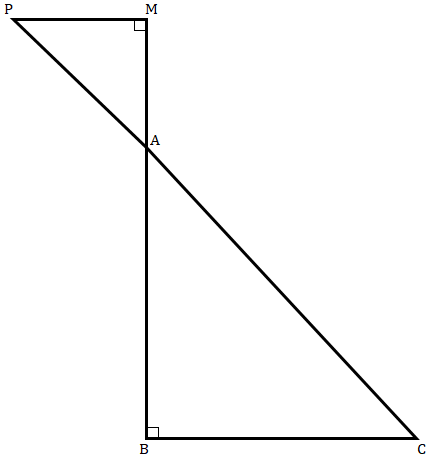"
">

# If $őĒ\ ABC$ and $őĒ\ AMP$ are two right triangles, right angled at $B$ and $M$, respectively such that $‚ą†MAP\ =\ ‚ą†BAC$. Prove that:(i) $őĒABC\ ‚ąľ\ őĒAMP$(ii) $\frac{CA}{PA}\ =\ \frac{BC}{MP}$"

Given:

$Δ\ ABC$ and $Δ\ AMP$ are two right triangles, right-angled at $B$ and $M$, respectively such that $∠MAP\ =\ ∠BAC$.

To do:

We have to prove that:

(i) $ΔABC\ ∼\ ΔAMP$

(ii) $\frac{CA}{PA}\ =\ \frac{BC}{MP}$

‚ÄäSolution:

(i) In $\vartriangle AMP$ and $\vartriangle ABC$,

$\angle AMP = ∠ABC = 90^o$

$\angle MAP = \angle BAC$   (Vertically opposite angles)

Therefore,

$\vartriangle ABC ∼ \vartriangle AMP$     (By AA similarity)

(ii) $\vartriangle ABC ∼ \vartriangle AMP$

This implies,

$\frac{CA}{PA} = \frac{BC}{MP}$  (Corresponding sides are proportional)

Hence proved.

Updated on: 10-Oct-2022

17 Views